# Wegener's Mirage Model

## Wegener's model

The simplest model for superior mirages is Alfred Wegener's model, as elaborated in his 1918 paper. It reduces the inversion layer to a temperature discontinuity. Of course, this is completely unphysical; still, it captures some basic behavior, so it's useful heuristically. Just remember that this involves an incorrect notion: that the mirage is produced by total internal reflection.

I'll translate a little of what Wegener says about his model into English:

A mirage can first appear if the angle of incidence exceeds the limiting value for total reflection. Then, as seen by the observer, the mirage must be restricted to a strip of definite width, whose center lies exactly in the astronomical horizon.

He then points out that the limiting value of the incidence angle restricts all ducted rays to a spherical shell concentric with the surfaces of constant density (or temperature) in the atmosphere. Now comes the section of interest to us here: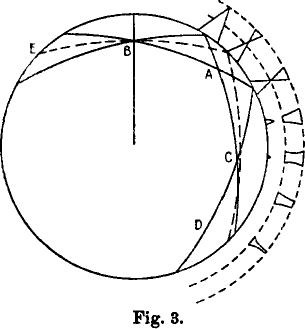Some things about the influence of distance on the form of the reflected images can be obtained from Fig. 3. In it are drawn the two limiting rays of total reflection and the horizontal ray (the latter dashed) from the observer at B. If one calculates the backward extensions to E, the two limiting rays are obviously equally long; but the horizontal ray is shorter.

Consequently, the two points of reflection of the limiting rays at E, A and D must also always be equally far apart, while the reflection point of the horizontal ray is shifted back more at each new reflection. The path of the rays is therefore not exactly symmetric at the reflection point A, and at C only the two limiting rays intersect, while the horizontal ray here runs somewhat above this intersection. But still symmetry is preserved enough that all totally reflected rays, which fill an appreciable space at A, are drawn together at C like a knot. Thus BC is that distance at which the object has the greatest vertical exaggeration; for an object of small height that is found here fills the whole width of the reflecting strip for the observer. On the other hand, the least distortion of the mirror image is to be expected just beyond A, reckoned from the observer. Also, between C and D the distortion decreases again; though it still persists here in the upper part of the mirror image up to the reflection point. In the figure, the direct and reflected images are schematically explained for the example of a steep pyramid: While the erect image sinks more and more below the horizon with increasing distance from the observer, the mirror images are bound to the totally reflecting strip, which always lies at the same height. The reflection first begins in the upper part of this strip; with increasing distance the mirror image extends downward, so that the peak of the pyramid finally would lie below the reflecting strip and is therefore invisible. At the maximal phase of vertical distortion, occurring at C, an interesting phenomenon now appears: beyond it, the mirror image appears erect again. Thus the vertical distortion produces a continual transition from inverted to erect mirror images. Thereby the presence of this vertical distortion, which plays so great a role in mirages, is explained.

The diagram is a little confusing, because the circle serves double duty: first, as the reflecting density discontinuity in the atmosphere (for the rays drawn inside it); and second, as the surface of the Earth (for the images of the pyramid, drawn outside it). The observer is assumed to be high enough above the surface to see a considerable width of undistorted images below the strip that contains the miraged images.

The miraged strip is indicated in the images outside the circle by a pair of dashed circular arcs. Notice that Wegener generally calls this zone a “reflecting strip” here, rather than a “blank strip” — a term he introduced later in the same paper, when discussing sunset phenomena.

If you still find Wegener's diagram uninformative, try my attempt to explain his ideas with simpler diagrams.

## Checking Wegener's sketch

In the 90 years that have elapsed since Wegener's paper was published, computers have made it possible to do an accurate calculation for his model. As he made no attempt to be quantitative in drawing the figure above, and qualifies it as schematic, the height of the observer is rather arbitrary. Furthermore, no value is offered for the temperature jump at his zero-thickness inversion layer; so several important parameters are left open.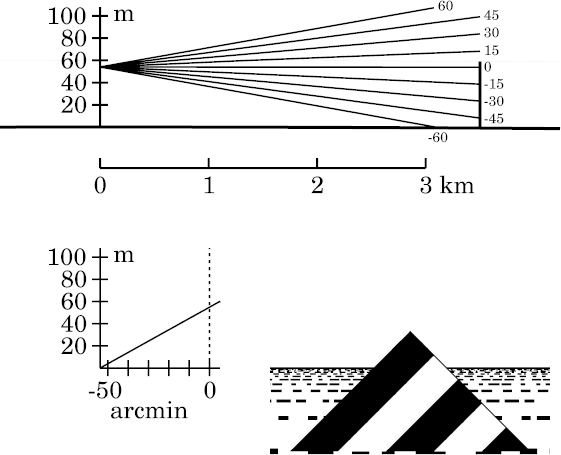However, it's clear from his drawing and the accompanying explanation that his pyramidal target just reached to the height of the discontinuity that represents the inversion. So let's try collapsing our usual 2° inversion to a very thin layer at its top, 60 m above the ground, and choose a triangular target of that same height (60 m), instead of the usual 200-m target. As the observer must be somewhere in the duct, let's try the 54-m height of the eye used on a previous page.

### Target at 3.5 km

At the left here are the ray diagram, transfer curve, and target appearance for Wegener's model when the target is 3.5 km from the eye, which corresponds to Wegener's first drawing of his pyramid, at the top of his figure. This is just inside the range at which miraging (image inversion) first appears.

The target, which (as the simulation shows) is well inside the visible horizon, appears practically undistorted.

Note that the inversion, or discontinuity, is very dimly visible as a pale gray line at 60 m above the surface, touching the top of the target in the ray diagram. (As it effectively has zero thickness, there's no gray band here marking the inversion, as there is on the pages for more realistic models.)

### Target at 10 km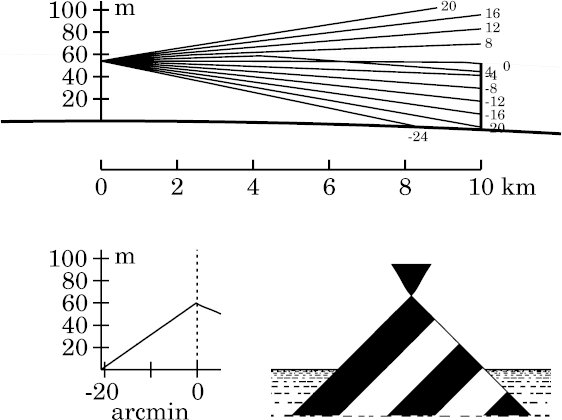Now let's move the target out to 10 km. Here the classical superior mirage is plainly visible; this corresponds approximately to Wegener's second sketch, showing the miraged peak of the pyramid, in his figure.

That's as Wegener predicted. But he drew the miraged point of the pyramid with the same apex angle as that of the erect image below it — while we see here that the miraged (reflected) image is stretched vertically: the apex angle of the inverted image is quite acute, instead of a right angle. That's because the reflection takes place at a concave surface, not a flat one. And a concave mirror is a magnifying mirror — all the more so, at grazing incidence. Wegener overlooked this magnification.

Notice that the ray at 4′ of arc above the observer's astronomical horizon was reflected where it met the discontinuity, a little more than 4 km from the observer. This ray intersects the observer's horizon ray (at 0′) between 6 and 7 km from the eye. This ray crossing, of course, is required to produce the inverted image of the mirage.

The corner in the transfer curve, just below the astronomical horizon, separates the erect and inverted images. It corresponds to a ray that is reflected by Wegener's discontinuity just at the distance of the target; because his target is the same height as the discontinuity, that's also the image of the target's peak. The slopes of the two segments of the transfer curve are inversely proportional to the magnifications of the two parts of the image: the segment with negative slope corresponds to the inverted part of the image. (Notice that the horizon ray is reflected just before the target; so it belongs to the inverted part of the image.)

### Target at 16 km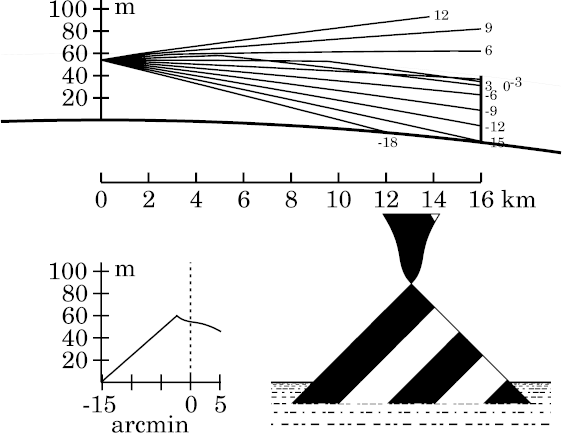With increasing distance, another effect predicted by Wegener appears: the distortion of the reflected (inverted) image. However, we find that it's a bit more complicated than he indicated in his figure.

Here, at 16 km distance, the inverted image of the simple superior mirage is becoming vase-shaped. That's because the magnification of the image varies with distance. The transfer curve shows that the magnification is greatest near the astronomical horizon; that produces the stretching in the middle part of the vase.

Of course, this effect can be traced directly to Wegener's rule that the observer's line of sight meets the reflecting surface overhead at the maximum angle of incidence at the astronomical horizon. (That maximizes the foreshortening, and hence the magnifying power, of the concave reflector.) The symmetry of that maximum explains the symmetry of the “reflecting strip” about the astronomical horizon. (This effect is discussed further on another page, in connection with Cassini's refraction model.)

The flat top on the vase here, like the flat top on the inverted image in the previous figure, is the upper edge of Wegener's strip. (Its lower edge isn't visible at this target distance.)

The corner in the transfer curve that separates the erect and inverted images is more than 3′ below the astronomical horizon here. Because of the distortion of the inverted part, this corner is now a cusp. (As before, it marks a ray that meets Wegener's discontinuity at the target distance, and so is the image of the top of his target.)

Despite the distortion of the inverted image, we still have just two images of the miraged parts of the target. So this would be a 2-image superior mirage.

### Target at 22 kmAt 22 km, the “vase” has developed a waist, and resembles a dart. The part between the waist and the bulge below it is, of course, a vertically magnified, erect image of a small piece of the target, near its top.

This erect image, as predicted by Wegener in discussing his figure, is caused by the confluence of ducted rays “like a knot”, between 15 and 20 km from the observer. Here, all the rays within 4′ of the observer's astronomical horizon are roughly focused into a small part of the target; so that small part appears expanded vertically to fill this 8′ interval. The parts of the target that are optically conjugate to the observer's eye are infinitely stretched, producing vertical edges in the miraged image about 2′ above and below the astronomical horizon.

But the parts of the target less than 2′ from the astronomical horizon lie beyond their corresponding ray-crossings (about 20 km from the observer). This ray-crossing alone would make the observer see a real, inverted image of those parts; but that inverted image is inverted once again by the reflection of these rays at Wegener's density discontinuity about 10 km from the observer, producing another ray-crossing, and hence an erect image. As you would expect (cf. the transfer curve), this erect part of the image is centered on the observer's astronomical horizon.

The smooth transitions here between erect and inverted sections of the mirage are best thought of as examples of image-folding. Notice that these folds in the image are smooth, even though the temperature profile was discontinuous at 60 m height. The smoothness results from the continuous change in ray length as we look farther from the astronomical horizon; this shifts the image inversion that occurs at the ray-crossing smoothly through the position of the target.

The infinite vertical magnification at the image folds, and the generally great vertical magnification of the miraged part of this image, suggest how the Fata Morgana is formed. (Notice that the parts of the target in the erect image near the astronomical horizon are imaged four times here.)

The cusp in the transfer curve again marks the ray that meets Wegener's discontinuity (at the top of the target) at the target distance: cf. the −4′ ray in the ray diagram.

### Target at 30 km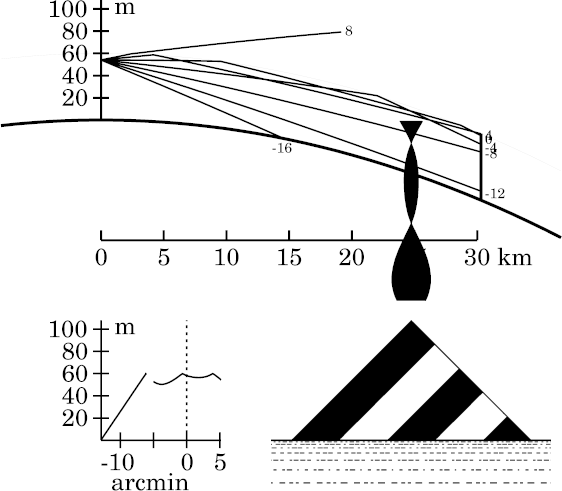If we move the target still farther away, the lower edge of Wegener's strip finally truncates the inverted tip of the miraged pyramid, leaving a gap between the inverted superior mirage and the direct image below it, as he predicted, The lower edge of the strip can be regarded as a superior mirage of the upper edge.

Here, the full width of the miraging strip is visible — from about 5′ above the astronomical horizon to 5′ below it (see the transfer curve).

The transfer curve now has two cusps. Each marks a double image (erect and inverted) of the top of the target. The cusp at 4′ above the astronomical horizon corresponds to a ray reflected at Wegener's discontinuity about 4 km from the observer; it just reaches the discontinuity (and hence the top of Wegener's target) again at the target. The second cusp, just below the astronomical horizon, corresponds to a ray reflected twice: once about 10 km from the observer, and then again a few km before the target. (This ray is not shown; but the ray at the astronomical horizon gives a good idea of where it goes.)

This double reflection illustrates Wegener's discussion of the relative intervals between reflections of rays at the horizon and at the edges of the miraged strip. Rays near the astronomical horizon are reflected at shorter intervals than rays near the edges of the strip, so there is room for two reflections of rays near the horizon between observer and target, but only one for rays with greater inclinations. (I have a more detailed discussion of this principle on another page.)

Furthermore, as reflections at Wegener's discontinuity alternate with ray-crossings between reflections, and both produce image inversions, we see that the number of image inversions increases from the edges of the strip (which have only a single inversion, and hence an inverted image) to its center. So we see two inversions, and hence an erect image, near altitudes of ±3 ′; but 3 inversions (an inverted image again) at the astronomical horizon itself (just above the lower cusp).

Hence, we see as many as 5 or 6 images of some miraged regions. These multiple images would easily be visible to the naked eye. This suggests that the Fata Morgana is simply a superior mirage of a sufficiently distant object. In terms of Wegener's simple reflections, it requires multiple reflections of the rays at the top of the duct.

Wegener's insight into the relation between the interval between successive reflections along different rays and the distortions in superior mirages is also useful for understanding the effect of eye height on these mirages.

## Final remarks

Wegener's “toy” mirage model already shows more complex behavior than he had expected. However, it's too unrealistic to be used to interpret actual superior-mirage displays. To understand the real world, we need to use a more realistic model of the inversion to generate the simulations.